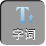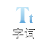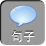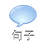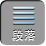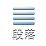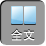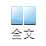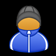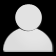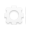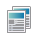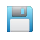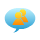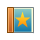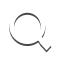-AA+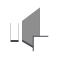1、计算 8.5 -( -1.5)

2、计算(－ 6)+ 9－(－ 1)－ 16+(－ 2).

＝(+ 3)+(+ 1)+(－ 16)+(－ 2)

＝(+ 4)+(－ 16)+(－ 2)

＝(－ 12)+(－ 2)

＝－ 14.

3、计算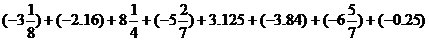.

＝[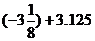]+[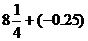]+[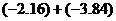]+[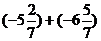]

0+( -6)+ 8+( -12)

-10.